Tamilnadu State Board New Syllabus Samacheer Kalvi 11th Computer Science Guide Pdf Chapter 12 Arrays and Structures Text Book Back Questions and Answers, Notes.

## Tamilnadu Samacheer Kalvi 11th Computer Science Solutions Chapter 12 Arrays and Structures

### 11th Computer Science Guide Arrays and Structures Text Book Questions and Answers

Book Evaluation

Part I

Question 1.
which of the following is the collection of variables of the same type that are referenced by a common name ?
a) int
b) float
c) Array
d) class
c) Array

Question 2.
Array subscripts is always starts with which number ?
a) -1
b) 0
c) 2
d) 3
b) 0Question 3.
int age[ ]={6,90,20,18,2}; How many elements are there in this array?
a) 2
b) 5
c) 6
d) 4
b) 5

Question 4.
cin>>n; To which element does this statement accepts the value?
a) 2
b) 3
c) 4
d) 5
c) 4

Question 5.
By default, the string end with which character?
a) \0
b) \t
c) \n
d) \b
a) \0Part – II

Question 1.
What is Traversal in an Array?
Accessing each element of an array at least once to perform any operation is known as “Traversal”. Displaying all the elements in an array is an example of “traversal”.

Question 2.
What is Strings?
A string is defined as a sequence of characters where each character may be a letter,’ number or a symbol.
Every string is terminated by a null (\0) character which must be. appended at the end of the string.

Question 3.
What is the syntax to declare two – dimensional array? ‘
The declaration of a 2-D array is
datatype array_name[row – size] [col – size];
In the above declaration, data-type refers to any valid C++ data – type, array _ name refers to the name of the 2 – D array, row – size refers to the number of rows and col-size refers to the number of columns in the 2 – D array.Part – III

Question 1.
Define an Array. What are the types?
An array is a collection of variables of the same type that are referenced by a common name.
There are different types of arrays used in C++. They are:

• One-dimensional arrays
• Two-dimensional arrays
• Multi-dimensional arrays

Question 2.
With note an Array of strings.
An array of strings is a two – dimensional character array. The size of the first Index (rows) denotes the number of strings and the size of the second index (columns) denotes the maximum length of each string. Usually, an array of strings are declared in such a way to accommodate the null character at the end of each string. For example, the 2-D array has the declaration:
char name ;
In the above declaration,
No. of rows = 7;
No. of columns = 10;
We can store 7 strings each with a maximum length of 10 characters.Question 3.
Write a C++ program to accept and print your name?
PROGRAM
#include<iostream>
using namespace std;
int main( )
{
char str;
cin.get(str,100);
cout<< “You name is : ” << str <<endl;
return 0;
}
Output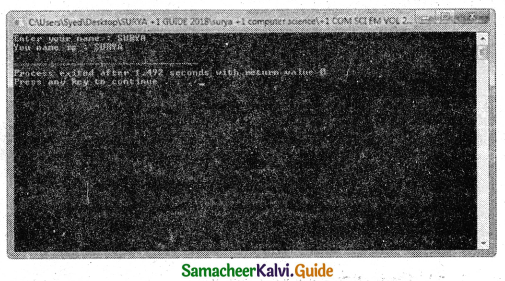Part – IV

Explain In Detail

Question 1.
write a c++ program to find the difference between two matrix.
PROGRAM
#include<iostream>
#include<conio.h>
using namespace std;
int main( )
{
int row, col, m1, m2, sum;
cout<<“Enter the number of rows : “;
cin>>row;
cout<<“Enter the number of columns :”;
cin>>col;
cout<< “Enter the elements of first matrix: “<<endl;
for (int i = 0;i<row;i++ )
for (int j = 0;j <col;j++ )
cin>>m1[i][j];
cout< < “Enter the elements of second matrix: “<<endl;
for (int i = 0;i<row;i++ )
for (int j = 0;j<col;j++ )
< cin>>m2[i][j];
cout<<“Output: “<<endl;
for (int i = 0;i<row;i++ )
{
for (int j = 0;j<col;j++ )
{
sum[i][j]=m1[i][j] – m2[i][j]); cout<<sum[i][j]<<“”;
}
cout<<endl<<endl;
}
getch( );
return 0;
}
Output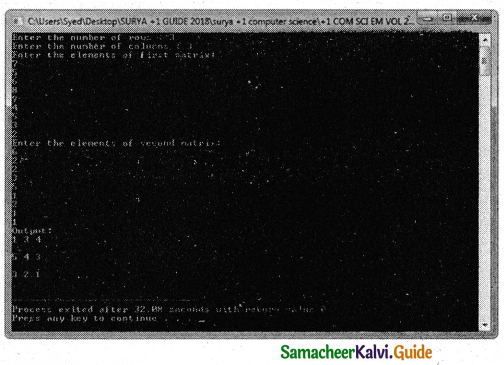Question 2.
How will you pass two dimensional array to a function explain with example.
Passing 2″D array to a function:
C++ program to display values from two dimensional array.
#include<iostream>
using namespace std;
void display (int n);
int main( )
{
int num = { {3, 4}, {9, 5>, {7, 1} } ;
display(num);
return 0;
}
void display(int n)
{
cout << “\n Displaying Values” << endl; for (int i=0; i<3; i++)
{
for (int j=0; j<2; j++)
{
cout << n[i][j] << “”;
}
cout << endl << endl;
}
}
Output
Displaying Values
3 4
9 5
7 1
In the above program, the two-dimensional array num is passed to the function display
( ) to produce the results.### 11th Computer Science Guide Arrays and Structures Additional Questions and Answers

Choose The Correct Answer (1 Mark)

Question 1.
The size of the array is referred to as its ………………..
(a) dimension
(b) direction
(c) location
(d) space
(a) dimension

Question 2.
…………… is an easy way of storing multiple values of the same type referenced by a common name.
a) Variables
b) Literals
c) Array
d) None of these
c) Array

Question 3.
Displaying all the elements in an array is an example of ………………..
(a) memory allocation
(b) call by reference
(c) traversal
(d) none of these
(c) traversalQuestion 4.
A (n) ………………….. is a collection of variables of the same type that are referenced by a common name.
a) Structures
b) Array
c) Unions
d) None of these
b) Array

Question 5.
During ……………….. the array of elements cannot be initialized more than its size.
(a) declaration
(b) initialization
(c) assigning
(d) execution
(b) initialization

Question 6.
The …………….. of the array is referred to as its dimension.
a) Elements
b) Size
c) Format
d) None of these
b) SizeQuestion 7.
Pass an array to a function in C++, the function needs the array name as ………………..
(a) a function
(b) an argument
(c) global object
(d) string
(b) an argument

Question 8.
…………………. is a type of arrays used in C++.
a) One-dimensional array
b) Two-dimensional array
c) Multidimensional array
d) All the above
d) All the above

Question 9.
A structure without a name tag is called ………………..
(a) homogenous structure
(b) anonymous structure
(c) array of structure
(d) dynamic memory
(b) anonymous structureQuestion 10.
A one-dimensional array represents values that are stored in a single …………….
a) Row
b) Column
c) Either A or B
d) None of these
c) Either A or B

Question 11.
Array size should be specified with …………………
a) square brackets[ ]
b) Parenthesis ( )
c) Curly braces{ }
d) Angle brackets < >
a) square brackets[ ]

Question 12.
In an array declaration, ……………… defines how many elements the array will hold.
a) array_name
b) array_size
c) data_type
d) None of these
b) array_sizeQuestion 13.
In an array declaration, …………………. declares the basic type of the array, which is the type of each element in the array.
a) array_name
b) array_size
c) data type
d) None of these
c) data type

Question 14.
In an array declaration, ……………….. specifies the name with which the array will be referenced
a) array_name
b) array_size
c) data type
d) None of these
a) array_name

Question 15.
The array subscript always starts with ………………
a) 0
b) 1
c) -1
d) None of these
a) 0Question 16.
The subscript always is a(n) ………………value.
a) Integer
b) Unsigned integer
c) Signed integer
d) float
b) Unsigned integer

Question 17.
In Turbo C++, int data type requires …………….. bytes of memory.
a) 4
b) 8
c) 2
d) 1
c) 2

Question 18.
In Dev C++, int data type requires ………………. bytes of memory,
a) 4
b) 8
c) 2
d) 1
a) 4Question 19.
The memory space allocated for an array can be calculated using the -formula.
a) Number of bytes allocated for the type of array + Number of elements
b) Number of bytes allocated for the type of array x Number of elements
c) Number of bytes allocated for the type of array – Number of elements
d) None of these
b) Number of bytes allocated for the type of array x Number of elements

Question 20.
An array can be initialized at the time of its ………………
a) Declaration
b) Process
c) Either A or B
d) None of these
a) Declaration

Question 21.
Unless an array is initialized, all the array elements contain ……………..values.
a) Null
b) Default
c) Garbage
d) None of these
c) GarbageQuestion 22.
While declaring and Initializing values In an array, the values should be given within the ……………..
a) square brackets[ ]
b) Parenthesis ()
c) Curly braces{ }
d) Angle brackets < >
c) Curly braces{ }

Question 23.
The …………….. of an array may be optional when the array is initialized during declaration.
a) Data type
b) Size
c) Name
d) None of these
b) Size

Question 24.
The subscript in the bracket can be a(n) ……………….
a) Variable
b) Constant
c) Expression that evaluates to an integer
d) Either A or B or C
d) Either A or B or CQuestion 25.
Accessing each element of an array at least once to perform any operation is known as ………………..
a) Traversal
b) Process
c) Reference
d) None of these
a) Traversal

Question 26.
…………………. is a process of finding a particular value present in a given set of numbers.
a) Filtering
b) Searching
c) Seeking
d) None of these
b) Searching

Question 27.
The ………………. compares each element of the list with the value that has to be searched until all the elements in the array have been traversed and compared.
a) Linear search
b) Sequential search
c) Either A or B
d) None of these
c) Either A or BQuestion 28.
A ……………. is defined as a sequence of characters where each character may be a letter, number, or a symbol.
a) String
b) Character
c) Literal
d) Identifier
a) String

Question 29.
In C++, there is no basic data type to represent a …………….
a) String
b) Character
c) Literal
d) float
a) String

Question 30.
How much memory required for the following array?
char country;
a) 12 bytes
b) 6 bytes
c) 60 Bytes
d) 24 bytes
b) 6 bytesQuestion 31.
…………….. is a way of initializing the character array:
a) char country={T, ‘N’ ‘D’ ‘I’, ‘A’, ‘\0’};
b) char country[ ]=”INDIA”;
c) char country[ ]={T, ‘N’, ‘D’, T, ‘A’ ‘\0’};
d) Either A or B or C
d) Either A or B or C

Question 32.
Which is a correct statement from the following?
a) At the end of the string, a null character is automatically added by the compiler.
b) If the size of the array is not explicitly mentioned, the compiler automatically calculates the size of the array based on the number of elements in the list and allocates space accordingly.
c) In the initialization of the string, if all the characters are not initialized, then the rest of the characters will be filled with NULL.
d) All the above
d) All the above

Question 33.
In C++,………………. is used to read a line of text including blank spaces.
a) cin.get( )
b) cin
c) put( )
d) None of these
a) cin.get( )Question 34.
………………. function can read the characters till it encounters a newline character or a delimiter specified by the user.
a) getline( )
b) put
c) puts( )
d) None of these
a) getline( )

Question 35.
…………………. arrays are a collection of similar elements where the elements are stored in a certain number of rows and columns.
a) One dimensional
b) Two dimensional
c) Multidimensional
d) All the above
b) Two dimensional

Question 36.
In two dimensional arrays, …………………. size is compulsory.
a) Column
b) Row
c) Both A and B
d) None of these
a) ColumnQuestion 37.
In two dimensional arrays, …………….. size is optional.
a) Column
b) Row
c) Both A and B
d) None of these
b) Row

Question 38.
int A[4 j; How many elements are there in the array “A”?
a) 3
b) 4
c) 7
d) 12
d) 12

Question 39.
The two-dimensional array uses ………………. index values to access a particular element in it.
a) two
b) one
c) three
d) many
a) twoQuestion 40.
In a two dimensional array, the first index specifies the …………….. value.
a) Column
b) Row
c) Both A and B
d) None of these
b) Row

Question 41.
In a two dimensional array, the second index specifies the …………… value.
a) Column
b) Row
c) Both A and B
d) None of these
a) Column

Question 42.
The two-dimensional array can be viewed as a …………………
a) List
b) Matrix
c) Linear block
d) None of these
b) MatrixQuestion 43.
There are …………… types of 2-D array memory representations.
a) two
b) one
c) three
d) many
a) two

Question 44.
……………… is a type of 2-D array memory representation.
a) Row-Major order
b) Column-Major order
c) Either A or B
d) None of these
c) Either A or B

Question 45.
In row-major order, all the elements are stored ……………… in contiguous memory locations.
a) Column by Column
b) Row by Row
c) Both A and B
d) None of these
b) Row by RowQuestion 46.
In column-major order, all the elements are stored ………………. in contiguous memory locations.
a) Column by Column
b) Row by Row
c) Both A and B
d) None of these
a) Column by Column

Question 47.
An array of strings is a ………………. dimensional character array,
a) Two
b) One
c) Multi
d) None of these
a) Two

Question 48.
In a two-dimensional character array, the size of the first index (rows) denotes the ……………….
a) Maximum length of each string
b) Number of strings
c) Maximum characters
d) None of these
b) Number of stringsQuestion 49.
In a two-dimensional character array, the size of the second index (rows) denotes the ……………….
a) Maximum length of each string
b) Number of strings
c) Maximum characters
d) None of these
a) Maximum length of each string

Question 50.
char Name; How many strings can be stored in the above array?
a) 10
b) 60
c) 6
d) 16
c) 6

Question 51.
To pass an array to a function in C++, the function needs the ………….. as an argument,
a) Array name
b) Array type
c) Dimension
d) None of these
a) Array nameQuestion 1.
What is the formula to calculate the memory space allocated for an array?
A number of bytes allocated for the type of array x Number of elements.

Question 2.
What are the types of arrays?
There are different types of arrays used in C++. They are:

1. One-dimensional arrays
2. Two-dimensional arrays
3. Multi-dimensional arrays

Question 3.
Write about returning structures from functions.
A structure can be passed to a function through its object. Therefore, passing a structure to a function or passing a structure object to a function is the same because the structure object represents the structure. Like a normal variable, a structure variable(structure object) can be passed by value or by references/addresses. Similar to built-in data types, structures also can be returned from a function.Question 4.
Write the syntax and example for one-dimensional declaration and initialization.
Syntax:
[size] = {value-1,value-2,………….,value-n};
Example: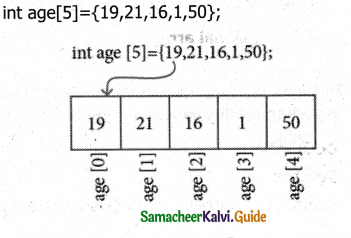Question 5.
What is a global object?
Objects declared along with structure definition are called global objects.

Question 6.
Write a note on strings.
strings:
A string is defined as a sequence of characters where each character may be a letter, number or symbol. Each element occupies one byte of memory. Every string is terminated by a null C\0′ ASCII code 0) character which must be appended at the end of the string.Question 7.
Differentiate array and structure.
Array:

• An array is a collection of variables of the same data type.
• Array data are accessed using index.
• Array allocates static memory
• Array element access takes lesser time.

Structure:

• A structure is a collection of variables of different data type.
• Structure elements are accessed using operator.
• Structures allocate dynamic memory.
• Structure elements take more time.

Question 1.
Write about the initialization of 2 – D array.
The array can be initialized in more than one way at the time of 2-D array declaration.
For example
int matrix = {
{10,20,30},// Initializes row 0
{40,50,60},// Initializes row 1
{70,80,90},// Initializes row 2
{100,110,120}// Initializes row 3
};
int matrix = {10, 20, 30, 40, 50, 60, 70, 80, 90, 100, 110, 120};
Array’s row size is optional but column size is compulsory.

Question 2.
What is subscript? Give its rules,
subscript:
Each element has a unique index number starting from 0 which is known as a subscript.
Rules for subscript:

• The subscript always starts with 0.
• It should be an unsigned integer value.
• Each element of an array is referred to by its name with a subscript index within the square bracket.

For example:
num refers to the 4th element in the array.Question 3.
How memory locations are allocated to a one-dimensional array?
Memory representation of a one-dimensional array:
The amount of storage required to hold an array is directly related to type and size.
The following figure shows the memory allocation of an array with five elements.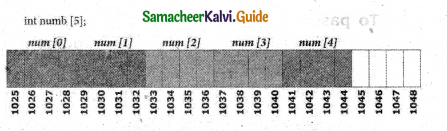The above figure clearly shows that the array num is an integer array with 5 elements. Here, there is a total of 5 elements in the array, where for each element, 4 bytes in Dev C++ (or)2 bytes in Turbo C++ will be allocated. Totally 20 bytes will be allocated for this array (In Turbo C++ 10 Bytes).

Question 4.
Tabulate the memory requirement of data type in Turbo C++ and Dev C++.

 Data type Turbo C++ Dev C++ char 1 1 int 2 4 float 4 4 long 4 4 double 8 8 long double 10 10Question 5.
Explain character Array (String) creation with syntax and example.
To create any kind of array, the size (length) of the array must be known in advance, so that the memory locations can be allocated according to the size of the array. Once an array is created, its length is fixed and cannot be changed during run time, This is shown in the following figure.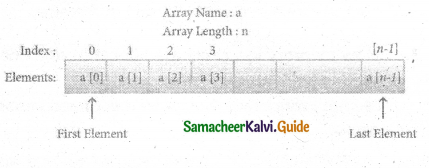Syntax of array declaration is:
char array_name[size];
In the above declaration, the size of the array must be an unsigned integer value.

For example,
char country;
Here, the array reserves 6 bytes of memory, for storing’ a seqi characters. The length of the string cannot be more than 5 characters and one location is reserved for the null character at the end.

Question 6.
What is an array of strings?
An array of strings is a two – dimensional character array. The size of the first index (rows) denotes the number of strings and the size of the second index (columns) denotes the maximum length of each string. Usually, an array of strings are declared in such a way to accommodate the null character at the end of each string. For example, the 2 – D array has the declaration: char Name;
In the above declaration, the 2 – D array has two indices which refer to the row size and column size, that is 6 refers to the number of rows, and 10 refers to the number of columns.

Question 7.
Write note on getline( ) function.
In C++, getline( ) is also used to read a line . of text from the input stream. It can read the characters tilt it encounters a newline character or a delimiter specifier’ by the user. This function is available in the header.Question 8.
How memory represented for a 2-D array?
There are two types of 2-D array memory representations. They are:

• Row Major order
• Column Major order

For example:
int A={ { 8,6,5}, { 2,1,9}, {3,6,4}, {4,3,2} };
Row Major order:
In row-major order, all the elements are stored row by row in continuous memory locations, that is, ail the elements in first row, then in the second row and soon. The memory representation of row-major order is as shown below.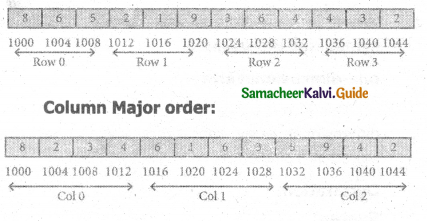Question 9.
How 2-D character array initialized and stored in memory? Explain.
2D char array initialization:

For example:
char Name = {“Mr. Bean’; “Mr.Bush”
“Nicole”, “Kidman” “Arnold”, “Jodie”};
In the above’ example, the 2-D array is initialized with 6 strings, where the string is a maximum of 9 characters long since the last character is null. The memory arrangement of a 2-D array is shown below and ail the strings are stored in continuous locations.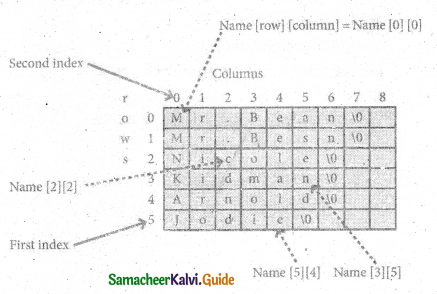Question 10.
What is called nested structure? Give example.
The structure declared within another structure is called a nested structure. Nested structures act as members of another structure and the members of the child structure can be accessed as parent structure name. Child structure name. Member name, struct dob
{
int date;
char month;
int year;
} ;
Values can be assigned to this structure as follows.
dob = {25, “DEC”, 2017}

Question 11.
Write a program to pass an array as an argument to a function.
In C++, arrays can be passed to a function as an argument.
To pass an array to a function in C++, the function needs the array name as an argument.
C++ program-to display marks of 5 students (one-dimensional array)
#indude<iostream>
using namespace std;
void display (int m);
int main( )
{
int marks={88, 76, 90, 61, 69};
display(marks);
return 0;
}
void’ display (int m)
{
cout << “\n. Display Marks: ” << end!; for (int i=0; i<5; i++)
{
cout <-< “Student” << i+1 << ” << m[i]<<endl;
}
}
Output
Display Marks:
Student 1: 88
Student 2: 76
Student 3: 90
Student 4: 61
Student 5: 69Question 12.
Write a C++ program to pass a 2-D array to a function.
C++ program to display values from two dimensional array:
#include<iostream>
using namespace std;
void display (int n);
int main( )
{
int num = { {3, 4}, (9, 5}, {7,1} };
display(num);
return 0; ‘
}
void display(int n)
{
cout << “\n Displaying Values” << endl;
for (int i=0; i<3; i++)
{
for (int j=0; j<2; j++)
{
cout << n[i][j] << ”
}
cout << endl << endl;
}
}
Output
Displaying Values
3 4
9 5
7 1

Question 13.
Write a user-defined function to return the structure after accepting value through the keyboard. The structure definition is as follows: struct Item{int item no; float price;};
item accept (item i)
{

cout << “\n Enter the Item No:”; cin >> i.no;
cout << “\n Enter the Item Price:”; cin >> i.price;
return i;

}Explain in Detail (5 Marks)

Question 1.
How will you search an element in a one-dimensional array? Explain linear search.
Searching in a one-dimensional array:
Searching is a process of finding a particular value present in a given set of numbers. The linear search or sequential search compares each element of the list with the value that has to be searched until all the elements in the array have been traversed and compared.

Program for Linear Search
#include<iostream>
using namespace std;
int Search(int arr[ ], int size, int value)
{
for (int i=0; <size; i++)
{
if (arr[i] == value)
return i; // return index value
}
return -1;
}
int main( )
{
int num, val, id;
for (int i=0; i< 10; i++) .
{
cout<<“\n Enter value” << i+1 <<“=”;
cin>>num[i];
}
cout<< “\n Enter a value to be searched: “;
cin>>val;
id=Search(num,10,val);
if(id==-1) .
else
cout<< “\n The value is found at the position” << id+1;
return 0;
}
The above program reads an array and prompts for the values to be searched. It calls the Search() function which receives array, size and value to be searched as parameters. If the value is found, then it returns the array index to the called statement; otherwise, it returns -1.Question 2.
Explain two-dimensional array with syntax and example.
Two-dimensional array:
Two-dimensional (2D) arrays are collections of similar elements where the elements are stored in a certain number of rows and columns. An example m x n matrix where m denotes the number of rows and n denotes the number of columns is shown in the following figure, int arr;
2D array conceptual memory representation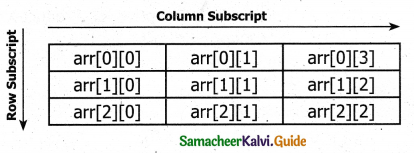The array arr can be conceptually viewed in matrix form with 3 rows and columns. point to be noted here is since the subscript starts with 0 arr  represents the first element.

The syntax for declaration of a 2-D array is:
data-type array_name[row-size][col-size];
In the above declaration, data-type refers to any valid C++ data-type, array_name refers to the name of the 2-D array, row-size refers to the number of rows and col-size refers to the number of columns in the 2-D array.

For example:
int A;
In the above example, A is a 2-D array, 3 denotes the number of rows and 4 denotes the number of columns. This array can hold a maximum of 12 elements.Case Study

Question (1)
Write a program to accept the marks of 10 students and find the average, maximum and minimum marks.
PROGRAM
#include<iostream>
using namespace std;
int main( )
{
int marks, sum=0,max,min;
float avg;
cout<< “\n Enter Mark 1” << “=”;
cin>> marks;
max=marks;
min=marks;
for(int i=1; i< 10; i++)
{
cout<< “\n Enter Mark” << i+1 << “=”;
cin>> marks[i];
sum=sum+marks[i];
if (marks[i]>max)
max = marks[i];
if (marks[i]<min)
min = marks[i];
}
avg=sum/10.0;
cout<< “\n The Total Marks: ” << sum;
cout<< “\n The Average Mark: ” <<avg;
cout<< “\n The Maximum Mark: ” <<max;
cout<< “\n The Minimum Mark: ” <<min;
}
Output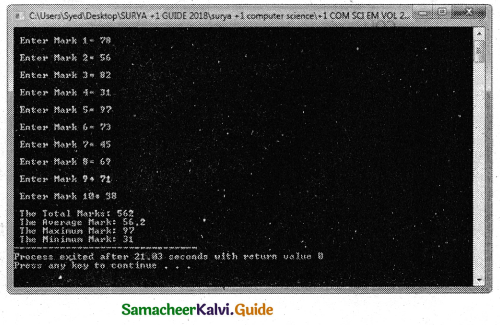Question (2)
Write a program to accept rainfall recorded in four metropolitan cities of India and find the city that has the highest and lowest rainfall.
PROGRAM
#include<iostream>
#include<string.h>
using namespace std;
int main( )
{
int rate,min,n;
char shop;
char minshop;
cout<<“\nHow many Shops
cin>>n;
for(int i=0;i<n;i++)
{
cout<<“\nEnter Name of the Shop “<<i+1<<” :”;
cin> >shop[i];
cout<<“\nEnter price of Product-X at “<<shop[i]<<” :”;
cin>>rate[i];
}
min=rate;
strcpy(minshop,shop);
for(int i=l;i<n;i++)
{
if (rate[i]<min)
{
min = rate[i];
strcpy(minshop,shop[i]);
}
}
cout< < “\n The Lowest cost of the Proudct=X at the” “<<minshop <<“is”<<min;
}
Output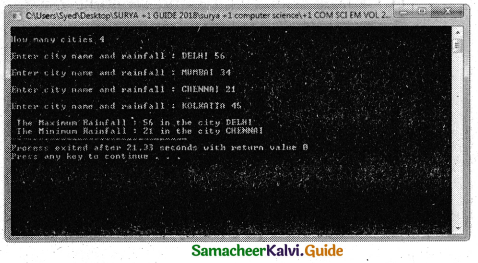Question (3)
Survey your neighboring shops and find the price of any particular product of interest and suggest where to buy the product at the lowest cost.
PROGRAM
#include<iostream>
#include<string.h>
using namespace std;
int main( )
{
int rate,min,n;
char shop;
char minshop;
cout<<“\nHow many Shops “;
cin>>n;
for(int i=0;i<n;i++)
{
cout<<“\nEnter Name of the Shop “<<i+l<<” :”;
cin>>shop[i];
cout<<“\nEnter price of Product-X at “<<shop[i]<<“:”;
cin> >rate[i];
}
min=rate;
strcpy(nninshop,shop);
for(int i=l;i<n;i++)
{
if (rate[i]<min)
{
min = rate[i];
strcpy(minshop,shop[i]);
}
}
cout< < “\n The Lowest cost of the Proudct=X at the ” <<minshop <<“is”<<min;
}
Output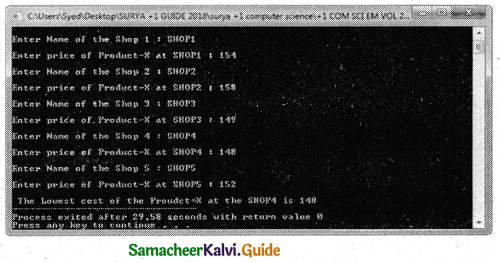STRUCTURES

Book Evaluation

Part -I

Question 1.
The data elements in the structure are also known as ……………..
a) objects
b) members
c) data
d) records
b) members

Question 2.
Structure definition is terminated by ……………..
a) :
b) }
c) ;
d) ::
c) ;

Question 3.
What will happen when the structure is declared?
a) it will not allocate any memory
b) it will allocate the memory
c) it will be declared and initialized
d) it will be only declared
b) it will allocate the memoryQuestion 4.
What is the out of this program?
#include<iostream>
#include<string.h>
using namespace std;
int main( )
{
struct student
{
int n;
char name;
};
students;
s.n = 123;
183
strcpy(s.name, “Balu”);
cout<<s.n;
co
ut<< s.name <<endl;
return 0;
}
a) 123Balu
b) BaluBalu
c) Balul23
d) 123 Balu
a) 123Balu

Question 5.
A structure declaration is given below.
struct Time
{
int hours;
int minutes;
int seconds;
}t;
Using the above declaration which of the following refers to seconds.
a) Time.seconds
b) Time::seconds
c) seconds
d) t. seconds
d) t. secondsQuestion 6.
What will be the output of this program?
#include <iostream>
using namespace std;
struct ShoeType
{
string name; double price;
>; , ‘ ‘
int main()
184 {
ShoeType shoe1, shoe2;
shoe1.price = 9.99;
cout<< shoe1.name<< ” #. “<< shoe1.
price<<endl;
shoe2 = shoe1;
shoe2.price = shoe2.price / 9;
cout<< shoe2.name<< ” # “<< shoe2.price;
return 0;Question 7.
Which of the following is a properly defined structure?
a) struct {int num;}
b) struct sum {int num;}
c) struct sum int sum;
d) struct sum {int num;};
d) struct sum {int num;};

Question 8.
A structure declaration is given below.
struct employee
{
int empno;
char ename;
} e;
Using the above declaration which of the following statement is correct.
a) cout<<e.empno<<e.ename;
b) cout<<e.empno<<ename;
c) cout< <e->empno< <e->ename;
d) cout<<e.empno<<e.ename;
a) cout<<e.empno<<e.ename;Question 9.
Which of the following cannot be a structure member?
a) Another structure
b) Function
c) Array
d) variable of double datatype
b) Function

Question 10.
When accessing a structure member, the identifier to the left of the dot operator is the name of
a) structure variable
b) structure tag
c) structure member
d) structure-function
a) structure variablePart – II

Question 1.
Define structure. What is its use?
Definition:
The structure is user-defined which has the combination of data items with different data types. This allows to a group of variables of mixed data types together into a single unit.
Use: The structure provides a facility to store different data types as a part of the same logical element in one memory chunk adjacent to each other.

Question 2.
To store 100 integer numbers which of the following is good to use?
Array or Structure State the reason.
In any situation when more than one variable is required to represent objects of uniform data types, an array can be used. If the elements are of different data types, then the array cannot support them.
The structure provides a facility to store different data types as a part of the same logical element in one memory chunk adjacent to each other. So, to store 100 integer numbers Array is good.

Question 3.
What is the error in the following structure definition.
struct employee {inteno; charename; char dept;}
Employee e1,e2;

Error:
In the above code objects for employee strcture is created at the end of structure definition. So,structure name is not needed. It may written as:
struct employee
{
int eno;
char ename;
char dept;
}e1,e2;Question 4.
Write a structure definition for the structure student containing exam no, name and an array for storing five subject marks.
Structure definition:
struct student
{
int examno;
char sname;
int mark ;
};

Question 5.
Why for passing a structure to a function call by reference is advisable to us?
In call by reference, any change made to the contents of structure variable inside the function are reflected back to the calling function. Otherwise changes in the structure content within the function will not implement in the structure content.Question 6.
What is the size of the following highlighted variable in terms of byte if it is compiled in dev C++
struct A{ float f; char ch;long double d;>;
struct B{ A a; int arr;}b
struct A {float f; char ch; long double d;>;
Memory requirements (Using Dev C++):

 Variable Memory requirement float f(3); 12 Bytes char ch;   , 5 Bytes double d; 8 Bytes

Using Turbo C++ also the same memory is needed. (Only int data type requirement is differ. Here no int members. So, no change in the requirement.)
struct B { A a; int arr;}b;
Memory requirements (Using Dev C++):

 Variable Memory requirement A a; (Which is a structure variable) 25 Bytes int arr; 24 Bytes b which is a structure variable of structure B 49 Bytes for each element of b. So, Total requirement of b is 147 Bytes.

Memory requirements (Using Turbo C++):

 Variable Memory requirement A a; (Which is a structure variable) 25 Bytes int arr; 12 Bytes b which is a structure variable of structure B 37 Bytes for each element of b. So, Total requirement of b is 111 Bytes.Question 7.
Is the following snippet is fully correct. If not identify the error.
struct sum1{ int n1,n2;}s1;
struct sum2{int n1,n2}s2;
cin>>s1.n1>>s1.n2;
s2=s1;
No. It is not fully correct. The following errors are there.
Errors:

 Statement Error struct sum1 {int n1,n2;}sl; No Error struct sum2 {int n1,n2}s2; Semicolon missing at the end of declaration statement; cin>>s1.n1>>s1. n2; No Error s2-s1; Objects of different structures can not be directly copied.

Question 8.
Differentiate array and structure.
In any situation when more than one variable is required to represent objects of uniform data¬types, array can be used. If the elements are of different data types, then array cannot support. If more than one variable is used, they can be stored in memory but not in adjacent locations. It increases the time consumption while searching.

The structure provides a facility to store different data types as a part of the same logical element in one memory chunk adjacent to each other.Question 9.
What are the different ways to initialize the structure members?
Values can be assigned to structure elements similar to assigning values to variables.
Example:
Consider the following structure: struct Student
{
long int rollno;
int age;
float weight;
}
balu ;
We can initialize as given below:
balu.rollno= 702016;
balu. age=18; ‘
balu.weight= 48.5;
Also, values can be assigned directly as similar to assigning values to Arrays.
balu={702016, 18, 48.5};

Question 10.
What is wrong with the following C++ declarations?
A. struct point ( double x, y)
B. struct point { double x, double y >;
C. struct point { double x; double y >
D. struct point { double x; double y; };
E. struct point { double x; double y; >

Errors:
A. struct point (double x, y)
Structure definition must be terminated with;. It is missing. Members must be enclosed in { }. So it may be written as
struct point { double x, y };

B. struct point {double x, double y>;
Structure member declaration must be terminated with; It is missing. So, it may corrected as
struct point {double x; double y;>; or struct point {double x, y;>;

C. struct point {double x; double y>
Structure member declaration and definition must be terminated with ; It is missing. So, it may corrected as
struct point {double x; double y;>; or
struct point { double x, y; >;

D. struct point {double x; double y;};,
No error .
E. struct point {double x; double y;>
– Structure definition must be terminated with ; it is missing. So, it may be corrected as
struct point {double x; double y;>;Part – III

Question 1.
How will you pass a structure to a function ?
A structure variable can be passed to a function in a similar way of passing any argument that is of built-in data type,

1. If the structure itself is an argument, then it is called “call by value”.
2. If the reference of the structure is passed as an argument then it is called, “call by reference”.

Call by value
When a structure is passed as argument to a function using call by value method,any change made to the contents of the structure variable inside the function to which it is passed do not affect the structure variable used as an argument.

Call by reference
In this method of passing the structures to functions, the address of a structure variable / object is passed to the function using address of(&) operator. So any change made to the contents of structure variable inside the function are reflected back to the calling function.Question 2.
The following code sums up the total of all students name starting with ‘S’ and display it. Fill in the blanks with required statements.
struct student {int exam no, lang, eng, phy, che, mat, esc, total; char name;>;
int main( )
{
student s;
for(int i=0;i<20;i++)
{
…………………. //accept student details
}
for(int i=0;i<20;i++)
{
……………. //check for name starts with’ letter “S”
…………….. // display the detail of the checked name
}
return 0;
}
MODIFIED PROGRAM
using namespace std;
#include<iostream>
struct student
{
int exam_.no,lang,eng,phy,che,mat,esc,total; char name;
};
int main( )
{
student s;
for(int i=0;i<20;i++)
{
//accept student details
cout<<“\nEnter student name”;
cin>>s[i].name;
cout<<“\nEnter student exam number”;
cin>>s[i].exam_no;
cout<<“\nEnter Languge Mark”;
cin>>s[i].lang;
cout<<“\nEnter Engjish Mark”;
cin>>s[i].eng;
cout<<“\nEnter Physics Mark”;
cin>>s[i].phy;
cout<<“\nEnter Chemistry Mark”;
cin>>s[i].che;
cout<<“\nEnter Maths Mark”;
cin>>s[i].mat;
cout<<“\nEnter Computer Science Mark”;
cin>>s[i].csc;
s[i].total = s[i].lang + s[i].eng + s[i]. phy+s[i].che+s[i].mat+s[i]. csc;
}
cout<<“\nDetails of student name starts with letter S “<<endl;
for(int i=0;i<20;i++)
{
//check for name starts with letter “S” if(s[i].name == ‘S’)
{
// display the detail of the checked name

cout<<“\nName : “<<s[i].
name<<“Total Mark”<<s[i].
total <<endl;
}
}
return 0;
}
Output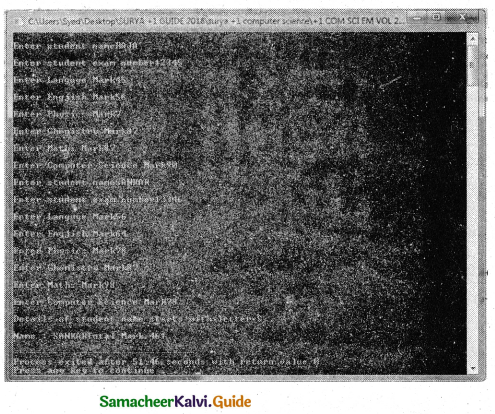Question 3.
What is called nested structure. Give example
The structure declared- within another structure is called a nested structure.
Example:
struct Student
{
int age;
float height, weight;
struct dob
{
int date;
char month;
int year;
};
}mahesh;
The nested structures act as members of another structure and the members of the child structure can be accessed as parent structure name. Child structure name. Member name.
Example:
mahesh. dob. date
Here

• mahesh is a parent structure name.
• dob is a child structure name
• date is a member of child structure

Question 4.
Rewrite the following program after removing the syntactical error(s), if any.
Underline each correction.
struct movie
{
charm_name;
charm-Lang;
float ticket cost =50;};
Movie;
void main( )
{
gets(m_name);
cin>>m-lang;
return 0; ,
}
MODIFIED PROGRAM
PROGRAM (USING DEV C++)
#include<iostream>
#include<conio.h>
#include<stdio.h>
struct movie
{
char m_name;
char m_lang;
float ticket_cost;
} Movie;
int main( )
{
std::cout<<“\nEnter Move! Name ;
gets(Movie.m_name);
std::cout<<:”\nEnter Movie Language ,:
std: :cin>>Movie.m -lang;
std::cout<<“\nEnter Ticket Cost: “;
std::cin>>Movie.ticket_cost;
std::cout<<“\nMovie name is : “<
std::cout< <“\nMovie Language is : “<<Movie.m-lang;
std::cout<<“\nTicket cost is : “<<Movie.ticket_cost;
}
Output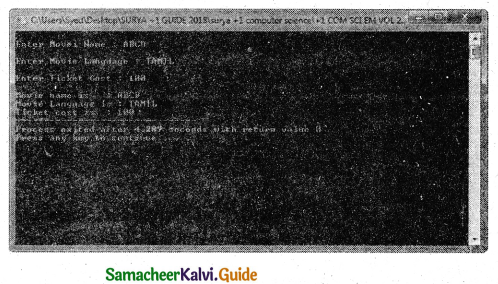Question 5.
What ¡s the difference among the following two programs?
a) #include<iostream.h>
struct point
{
double x;
double y;
};
int main()
{
struct point test;
testx = .25; test.y = .75;
cout< <test.x< ctest.y;
return 0;
}
b) #include
struct .
{
double x;
double y;
} Point;
int main(void)
{
Point test={.25,.75>;
return 0;
}
The method of structure initialisation is different.

Question 6.
How to access members of a structure? Give f example.
Once the two objects of student structure type are declared, their members can be accessed directly. The syntax for that is using a dot (.) between the object name and the member name.
Syntax is :
Object name. Member

For example:
struct Student
{
longrollno;
int age;
float weight;
}balu, frank;
The elements of the structure Student can be accessed as follows: balu.rollno balu.age balu. weight frank, rollno frank.age frank.weight
balu.rollno
balu.age
balu.weight
frank.rollno
frank.age
frank.weightQuestion 7.
Write the syntax and an example for structure.
Structure is declared using the keyword ‘struct’. The syntax of creating a structure is given below.
struct structure_name
{
type member_name1;
type member_name2;
} reference_name;
An optional field reference_name can be used to declare objects of the structure type directly.

Example:
struct Student
{
longrollno;
int age;
float weight;
}balu, frank;

Question 8.
For the following structure, definition writes the user-defined function to accept data through the keyboard.
struct date
{
int dd,mm,yy
};
struct item
{
int item id;
char name;
float price;
date date_manif;
}
USER DEFINED FUNCTION
item accept( )
{
item obj;
cout<<“\n Enter Item Id “;
cin> > obj. item id;
cout<<“\nEnter Name “;
cin>obj.name;
cout<<“\nEnter Price “;
cin>>obj.price;
cout<<“\nEnter Date: date, month and year
cin>>obj.date_manif.dd >> obj.date_manif. mm >> obj.date_manif.yy;
return(obj);
}Question 9.
What is called an anonymous structure? Give an example.
A structure without a name/tag is called anonymous structure, struct
{
long rollno;
int age;
float weight;
} student;
The student can be referred to as a reference name to the above structure and the elements can be accessed like
student, roll no
student.age and
student.weight.

Question 10.
Write a user-defined function to return the structure after accepting value through the keyboard. The structure definition is as follows:
struct Item{int item no;float price;};
USER DEFINED FUNCTION
Item accept( )
{
Item obj; ,
cout<<‘AnEnter Item Number and Price “; cin>>obj.item_no>>obj, price.
return(obj);
}Part-IV

Explain in Detail

Question 1.
Explain the array of structures with examples.
A class may contain many students. So, the definition of structure for one student can also be extended to all the students. If the class has 20 students, then 20 individual structures are required. For this purpose, an array of structures can be used.
An array of structures is declared in the same way as declaring an array with built-in data types like int or char.
The following program reads the details of 20 students and prints the same.
#include<iostream>
using namespace std;
struct Student
{
int age;
float height, weight;
char name;
};
void main()
{
Student std;
int i;
cout<< ” Enter the details for 20 students”<<endl;
for(i=0;i<20;i++)
{
cout<< ” Enter the details of student”<<i+1<<endl;
cout<< ” Enter the age:”<<endl; cin>>std[i].age;
cout<< “Enter the height:”<<endl; cin>>std[i].height;
cout<< “Enter the weight:”<<endl; cin>>std[i].weight;
}
cout<< “The values entered for Age, height and weight are”<<endl;
for(i=0;i<20;i++)
cout<< “Student “<<i+l<< “\t”< <std[i]. age<< “\t”<<std[i].height<< “\t”<<std[i]. weight;
}
Output
Enter the details of 20 students
Enter the details for student1
Enter the age:
18
Enter the height:
160.5
Enter the weight:
46.5
Enter the details for student2
Enter the age:
18
Enter the height:
164.5
Enter the weight:
61.5
………………………………….
…………………………
The values entered for Age, height and weight are
Student 1 18 160.5 46.5
Student 2 18 164.5 61.5
………………………Question 2.
Explain call by value with respect to structure.
A structure variable can be passed to a function in a similar way of passing any argument that is of built-in data type. If the structure itself is an argument, then it is called “call by value”.

Call by value
When a structure is passed as argument to a function using call by value method,any change made to the contents of the structure variable inside the function to which it is passed do not affect the structure variable used as an argument.
Example:
#include<iostream>
using namespace std;
struct Employee
{
char name; ,
int age;
float salary;
};
void printData(Employee); // Function declaration
int main( )
{
Employee p;
cout<< “Enter Full name: “;
cin>>p.name;
cout<< “Enter age: “;
cin>>p.age;
cout<< “Enter salary: “;
cin>>p.salary;
// Function call with structure variable as an argument
printData(p);
return 0;
}
void printData(Employee q)
{
cout<< “\nDisplaying Information.” <<endl;
cout<< “Name: ” << q.name <<endl;
cout<<“Age: ” <<q.age<<endl;
cout<< “Salary:” <<q.salary;
}
Output
Enter Full name: Kumar
Enter age: 55
Enter salary: 34233.4
Displaying Information.
Name: Kumar
Age: 55
Salary: 34233.4
In the above example, a structure named Employee is declared and used. The values that are entered into the structure are name, age and salary of a Employee are displayed using a function named printData( ).Question 3.
How call by reference is used to pass structure to a function .Give an Example
Call by reference
In this method of passing the structures to functions ,the address of a structure variable / object is passed to the function using address of(&) operator. So any change made to the contents of structure variable inside the function are reflected back to the calling function
Example:
#include<iostream>
using namespace std;
struct Employee
{
char name;
int age;
float salary;
};
void printData(Employee);
int main()
{
Employee p;
printData(p);
return 0;
}
{
cout<< “Enter Full name: “;
cin.get(p.name, 50);
cout<< “Enter age:
cin>>p.age; ,
cout<< “Enter salary:
cin>>p. salary;
}
void printData(Employee p)
{
cout<< “\nDisplaying Information.” <<endl;
cout<< “Name: ” << p.name <<endl;
cout<<“Age:” <<p.age<<endl;
cout<< “Salary:” <<p.salary;
}
Output
Enter Full name: Kumar
Enter age: 55
Enter salary: 34233.4
Displaying Information.
Name: Kumar
Age: 55
Salary: 34233.4
Structures are usually passed by reference method because it saves the memory space and executes faster.Question 4.
Write a C++ program to add two distances using the following structure definition
struct Distance
{
int feet;
float inch;
}d1, d2, sum;
PROGRAM
#include<iostream>
using namespace std;
struct Distance
{
int feet;
float inch;
} d1, d2, sum;
int main( )
{
cout<<“\nEnter distance 1: feet and inch : “;
cin>>d1.feet>>d1.inch;
cout<<“\nEnter distance 2: feet and inch :
cin>>d2.feet>>d2.inch;
sum.feet = d1.feet + d2.feet;
sum.inch =(d1.inch + d2.inch) – (int) (d1.inch + d2,inch) / 12 * 12;
sum.feet = sum.feet + (d1.inch + d2.inch)/12;
cout<<“\nDitance 1: Feet = “<<d1.feet<<”
Inch = “<<d1.inch;
cout<<“\nDitance 2: Feet = “<<d2.feet<<”
Inch = “<<d2.inch;
cout<<“\nSum of Distance 1 and 2 : Feet = “<<sum.feet<<” Inch = “<<sum.inch;
}
Output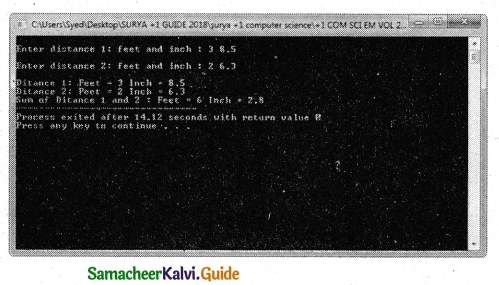Question 5.
Write a C++ Program to Add two Complex Numbers by Passing Structure to a Function for the following structure definition
struct complex
{
float real;
float imag;
};
The prototype of the function is complex add Complex Numbers(complex, complex);
PROGRAM
#include<iostream>
using namespace std;
struct complex
{
float real;
float imag;
};
complex add Complex Numbers (complex obj1,complex obj2)
{
complex obj3;
obj3.real = obj1.reai+obj2,real;
obj3.imag = obj1.imag + obj2.imag;
return(obj3);
}
int main( )
{
complex c1,c2,c3;
cout<<“\nEnter First complex number real and imaginary :”;
cin>>c1,real>>c1.imag;
cout<<“\nEnter Second complex number real and imaginary :”;
cin>>c2.real>>c2.imag;
cout<<“\nFirst Complex Number is : “< }
Output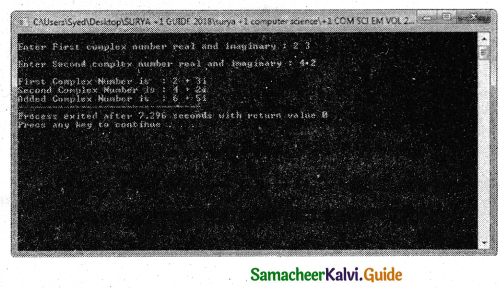Question 6.
WrIte a C++ Program to declare a structure book containing name and author as character array of 20 elements each and price as Integer. Declare an array of book. Accept the name, author, price detail for each book. Define a user defined function to display the book details and calculate the total price. Return total price to the calling function.
PROGRAM
using namespace std;
#include<iostream>
#include<iomanip>
#include<istdio.h>
struct book
{
char bname,author;
int price;
};
int display(book x)
{
int total;
for(int i=0;i<5;i++)
{
total = total + x[i].price;
cout<<“\nBook name : “<<x[i].
bname;
cout<<“\nAuthor name : “<<x[i].
author;
cout<<“\nPrice of the book: “<<x[i].
price;
}
return(total);C++C
}
int main( )
{
book. b;
for(int i=0;i<5;i++)
{
cout<<“\nEnter Book name “;
cin>>b[i].bname;
cout<<“\nEnter Author name “;
cin>>b[i].author;
cout<<“\nEnter Book Price “;
cin > > b[i]. price;
}
cout<<“\nDetails of Books”<<endl;
cout<<“\nTotal cost of the book is : Rs.”<<display(b);
}
Output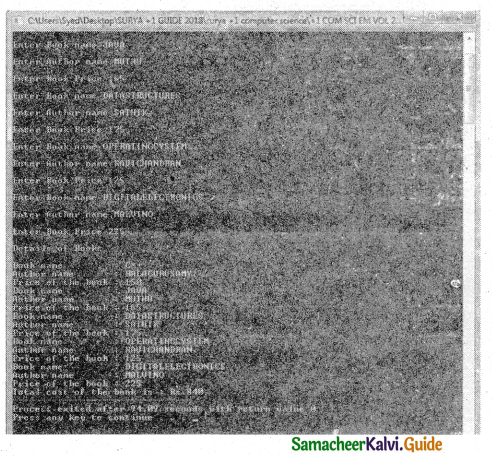Question 7.
Write a C++ program to declare and accept an array of professors. Display the details of the department=”COMP.SCI” and the name of the professors start with ‘A’. The structure “college” should contain the following members.
prof-id as integer ,
name and Department as character array
PROGRAM
using namespace std;
#include<iostream>
#include<iomanip>
#include<string.h>
struct college .
{
char pname,department; int prof-id;
};
void display(college x)
{
for(int i=0;i<5;i++)
{
if((strcmp(x[i]. department,”COMP. SCI”)==0) && x[i].pname==’A’)
cout<<x[i].pname«endl;
}
return;
}
int main( )
{
college c;
for(int i=0;i <5;i++)
{
cout<<“\nEnter Professor Name “;
cin>>c[i].pname; ,
cout<<“\nEnter Department Name “;
cin> >c[i] .department;
cout< <“\nEnter Professor Id
cin>>c[i].prof-id;
}
cout<<“\nDetails of Comp. Sci. . Dept, Professors whose name starts. with A “<<endl; . display(c);
}
Output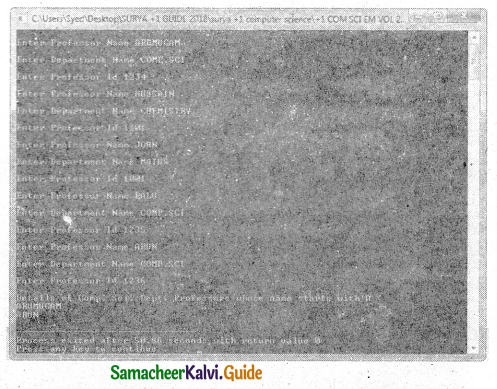Question 8.
Write the output of the following C++ program
#incluçle<iostream>
#include<stdio>
#include<string>
#include<conio>
using namespace std;
struct books
{
char name[20), author;
} a;
int main ( )
{
clrscr( );
cout<< “Details of Book No ” << 1 << “\n”;
cout<< “———————\n”;
cout<< ‘Book Name :”<<strcpy(a.
name,”Programming “)<<endl;
cout<< “Book Author :”<<strcpy(a.
author,”Dromy”)<<endl;
cout<< “\nDetails of Book No ” << 2 <<”\n”;
cout<< ” ——————- \n”;
cout<<- “Book Name :”<<strcpy(a[l].
name,”C++programming” )<<endl;
cout<< “Book Author :”<<strcpy(a[l].
author,”BjarneStroustrup “)< <endl;
cout<<“\n\n”;
cout<< “======================
============================
\n”; ‘
cout<< ” S.No\t| Book Name\t|author\n”;
cout<< “=======================
=============================
for (int i = 0; i < 2; i++)
{
cout<< “\n ” << i + 1 << “\t|” << a[i].name << “\t| ” << a[i],author;
}
cout<< “\n=========-==========
===========================
==”;
return 0;
}
Output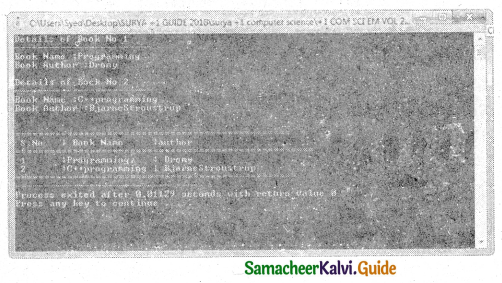Question 9.
Write the output of the following C++ program
#include<iostream>
#include<string>
using namespace std;
struct student
{
introll_no;
char name;
long phone_number;
};
int main() .
{
student p1 = {1,”Brown”,123443};
student p2, p3;
p2.roll_no = 2;
strcpy(p2.name,”Sam”);
p2.phone_number = 1234567822;
p3.roll_no = 3;
p3.phone_number = 1234567844;
cout<< “First Student” <<endl;
cout<< “roll no : ” << p1.roll_no <<endl;
cout<< “name : ” << p1.name <<endl;
cout<< “phone no ; ” << p1.phone_number <<endl;
cout<< “Second Student” <<endl;
cout<< “roll no : ” << p2.roll_no <<endl;
cout<< “name : ” << p2.name <<endl;
cout<< “phone no : ” << p2.phone_number <<endl;
cout<< “Third Student” <<endl;
cout<< “roll no : ” << p3.roll_no <<endl;
cout<< “name : ” << p3.name <<endl;
cout<< “phone no : ” << p3.phone_number <<endl;
return0;
}
Output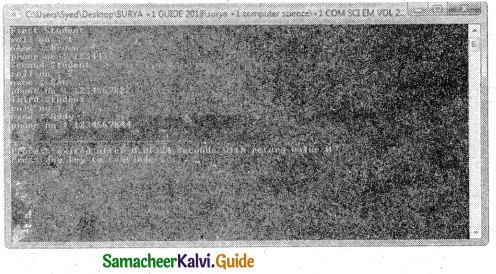Question 10.
Debug the error in the following program.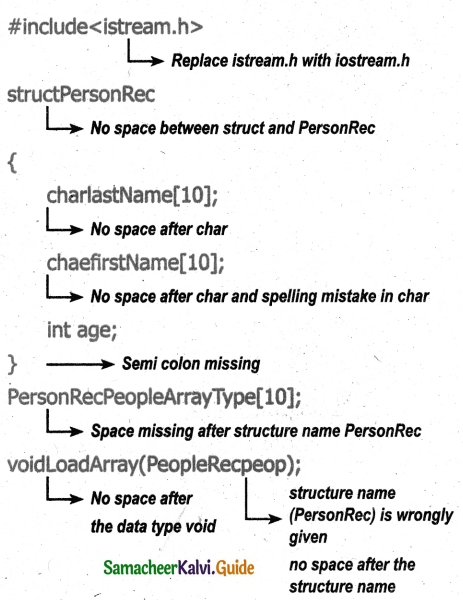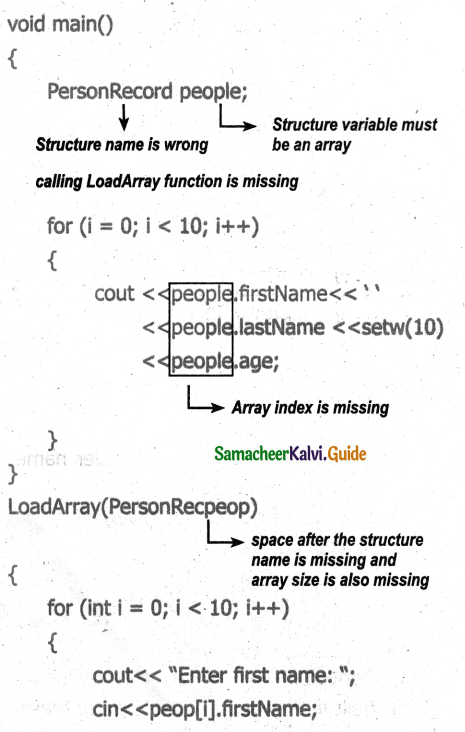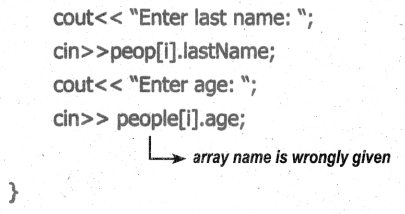MODIFIED PROGRAM
#include<iostream.h>
struct PersonRec
{
char lastName;
char firstName;
int age;
}
Person Rec PeopleArrayType[ 10];
void main( )
{
PersonRec people ;
for (i = 0; i < 10; i++)
{
cout< < people[i].firstName< < ” <<peopie[i].lastName <<setw(10) <<people[i].age;
}
}
{
for (int i = 0; i < 10; i++)
{
cout<< “Enter first name: “;
cin<<peop[i].firstName;
cout<< “Enter last name: “;
cin>>peop[i].lastName;
cout<< “Enter age: “;
cin>> peop[i].age;←
}### 11th Computer Science Guide Arrays and Structures Additional Questions and Answers

Choose The Correct Answer 1 mark

Question 1.
…………….. is a user-defined which has the combination of data items with different data types.
a) Structure
b) Class
c) Union
d) Array
a) Structure

Question 2.
…………………. allows to group of variables of mixed data types together into a single unit.
a) Structure
b) Class
c) Union
d) Array
a) Structure

Question 3.
If the elements are of different data types,then ……………… cannot support.
a) Structure
b) Class
c) Union
d) Array
d) ArrayQuestion 4.
Structure is declared using the keyword …………………
a) Structure
b) struct
c) Stru
d) STRUCT
b) struct

Question 5.
Identify the structure name from the following; struct Student balu;
a) balu
b) struct
c) Student
d) None of these
c) Student

Question 6.
Identify the structure variable (object) from the following: struct Student balu;
a) balu
b) struct
c) Student
d) None of these
a) baluQuestion 7.
How much of memory is required for the object of the following structure in Dev C++.
struct Student
{
long rollno;
int age;
float weight;
}balu, frank;
a) 12 Bytes
b) 8 Bytes
c) 10 Bytes
d) None of these
a) 12 Bytes

Question 8.
How much of memory is required for the object of the following structure in Turbo C++.
struct Student
{
long rollno;
int age;
float weight;
}balu, frank;
a) 12 Bytes
b) 8 Bytes
c) 10 Bytes
d) None of these
c) 10 BytesQuestion 9.
If the members are pointer types then ……………….. is used to access the structure members.
a) →
b) ←
c) .(dot)
d) None of these
a) →

Question 10.
A structure without a …………….. is called an anonymous structure.
a) Name
b) Tag
c) Name/Tag
d) None of these
c) Name/Tag

Question 11.
…………………… operator is used to accessing structure member.
a) ::
b) @
c) .(dot)
d) \$
c) .(dot)Question 12.
The structure declared within another structure is called a …………….. structure.
a) Nested
b) Group
c) Block
d) None of these
a) Nested

Question 13.
……………. structures act as members of another structure.
a) Nested
b) Group
c) Block
d) None of these
a) Nested

Question 14.
The members of the child structure can be accessed as …………………….
a) Child structure name. Parent structure name. Member name
a) Parent structure name. Child structure name. Member name
a) Parent structure name. Member name. Child structure name
a) Member name. Parent structure name. Child structure name
a) Child structure name. Parent structure name. Member nameQuestion 15.
A structure variable can be passed to a function in a similar way of passing any argument that is of ……………………. data type.
a) Derived
b) User-defined
c) Built-in
d) None of these
c) Built-in

Question 16.
If the structure itself is an argument to a function, then it is called ………………..
a) Call by value
b) Call by Reference
c) Call by Expression
d) None of these
a) Call by value

Question 17.
If the reference of the structure is passed as an argument then it is called ……………….
a) Call by value
b) Call by Reference
c) Call by Expression
d) None of these
b) Call by ReferenceQuestion 18.
When a structure is passed as an argument to a function using ……………… method, any change made to the contents of the structure variable inside the function to which it is passed do not affect the structure variable used as an argument.
a) Call by value
b) Call by Reference
c) Call by Expression
d) None of these
a) Call by value

Question 19.
In ……………….method of passing the structures to functions, any change made to the contents of structure variable inside the function are reflected back to the calling function.
a) Call by value
b) Call by Reference
c) Call by Expression
d) None of these
b) Call by Reference

Question 20.
Structures are usually passed by reference method because ……………..
a) It saves the memory space
b) Executes faster
c) Both A and B
d) None of these
c) Both A and BQuestion 21.
Identify the correct statement from the following.
a) A structured object can also be assigned to another structure object only if both the objects are of the same structure type.
b) The structure elements can be initialized either by using separate assignment statements or at the time of declaration by surrounding its values with braces.
c) Array of structure variable can also be created.
d) All the above
d) All the above

Question 1.
What is the drawback of an array?
In any situation when more than one variable is required to represent objects of uniform data-types, array can be used. If the elements are of different data types, then array cannot support.
If more than one variable is used, they can be stored in memory but not in adjacent locations. It increases the time consumption while searching.

Question 2.
What is the advantage of structure?
The structure provides a facility to store different data types as a part of the same logical element in one memory chunk adjacent to each other.

Question 3.
What are global objects?
Objects declared along with structure definition are called global objectsQuestion 4.
Write note on the anonymous structure.
A structure without a name/tag is called an anonymous structure.

Example:
struct
{
long rollno;
int age;
float weight;
} student;
The student can be referred to as a reference name to the above structure and the elements can be accessed like a student, roll no, student.age and student.weight

Question 5.
Define Nested structure.
The structure declared within another structure is called a nested structure.

Question 6.
Give an example of nested structure.
struct Student
{
int age;
float height, weight;
struct dob
{
int date;
char month;
int year;
};
}mahesh;Question 7.
How to access nested member structure?
The nested structures act as members of another structure and the members of the child structure can be accessed as
parent structure name. Child structure name. Member name
Example:
mahesh. dob. date

Question 1.
Write the syntax of defining a structure? Give an example.
Structure is declared using the keyword ‘struct’. The syntax of creating a structure is given below.
struct structure_name
{
type member-name1;
type member_name2;
} reference_name;
An optional field reference_name can be used to declare objects of the structure type directly.
Example:
struct Student
{
long rollno;
int age;
float weight;
};Question 2.
How memory is allocated for a structure?
Consider the following structure :
struct Student
{
long rollno;
int age;
float weight;
};
In the above declaration of the struct, three variables rollno, age and weight are used. These variables (data element) within the structure are called members (or fields). In order to use the Student structure, a variable of type Student is declared and the memory allocation is shown in the following figure.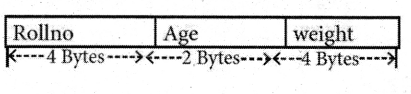Question 3.
How will you refer structure elements?
Referencing Structure Elements
Once the two objects of student structure type are declared, their members can be accessed directly.
The syntax for that is using a dot (.) between the object name and the member name. For example, the elements of the structure Student can be accessed as follows:
balu.rollno
frank, rollno
balu.age
frank.age
balu.weight
frank.weightExplain In Detail 5 Marks

Question 1.
Explain structure assignments in detail.